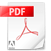## Chapter 3: Lattice vibration0. Introduction
Mineral Physics I 3. Lattice vibration 01. Boltzmann distribution
Mineral Physics I 3. Lattice vibration 1Student's work
3.1a.pdfStudent's work
3.1b.pdf2. Energy equipartition law and Dulong-Petit law
Mineral Physics I 3. Lattice vibration 2Student's work
3.2a.pdfStudent's work
3.2b.pdf3. One-dimensional quantum-mechanical harmonic oscillator
Mineral Physics I 3. Lattice vibration 3Student's work
3.3a.pdfStudent's work
3.3b.pdf4. Einstein model for heat capacity
Mineral Physics I 3. Lattice vibration 4Student's work
3.4a.pdfStudent's work
3.4b.pdf5. Phase and group velocities
Mineral Physics I 3. Lattice vibration 5Student's work
3.5a.pdfStudent's work
3.5b.pdf6. Dispersion relation
Mineral Physics I 3. Lattice vibration 67. Debye model
220109 Mineral Physics I 3. Lattice vibrStudent's work
3.7a.pdf